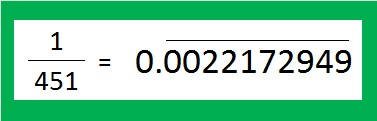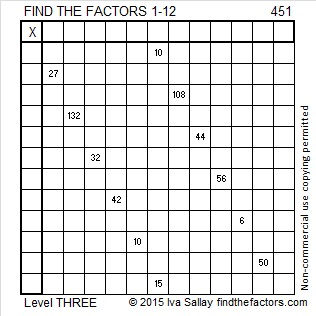# 451 and Level 3

Many fractions repeat a sequence of numbers forever when they are expressed as decimals:

• 1/3 in decimal form has an unending series of 3’s after the decimal point
• 1/11 is .090909… repeated forever. We say it has period two because exactly two digits repeat forever.
• 1/7 is .142857142857… repeated forever. It has period six because those six unique digits repeat forever.
• 1/451 has TEN digits repeat forever and ever. 451 is the smallest denominator whose decimal has ten digits repeating.Print the puzzles or type the factors on this excel file:  12 Factors 2015-04-06

——————————————————————————————————————-

• 451 is a composite number.
• Prime factorization: 451 = 11 x 41
• The exponents in the prime factorization are 1 and 1. Adding one to each and multiplying we get (1 + 1)(1 + 1) = 2 x 2 = 4. Therefore 451 has exactly 4 factors.
• Factors of 451: 1, 11, 41, 451
• Factor pairs: 451 = 1 x 451 or 11 x 41
• 451 has no square factors that allow its square root to be simplified. √451 ≈ 21.2368——————————————————————————————————————-

A Logical Approach to solve a FIND THE FACTORS puzzle: Find the column or row with two clues and find their common factor. Write the corresponding factors in the factor column (1st column) and factor row (top row).  Because this is a level three puzzle, you have now written a factor at the top of the factor column. Continue to work from the top of the factor column to the bottom, finding factors and filling in the factor column and the factor row one cell at a time as you go.